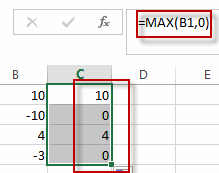# Changing Negative Number to Zero in Excel

This post will guide you how to change negative number to zero in a cell in Excel. How do I make negative numbers to zero with a formula in Excel. How to remove all negative numbers from a range of cells and then changed them to zero value in Excel.

## Changing Negative Number to Zero in Excel

If you want to change all negative numbers to zero value from a cell in Excel, you can use a formula based on the MAX function. Like this:

`=MAX(B1,0)`The MAX function will check if the value of the Cell B1 is less than 0, it indicated that this value is a negative value. And it will return 0. Otherwise, It will return the value of Cell B1.

You can also use another formula based on the IF function to change all negative numbers to zero in Excel. Just like this:

`=IF(B1<0,0,B1)`

### Related Functions

• Excel MAX function
The Excel MAX function returns the largest numeric value from the numbers that you provided. Or returns the largest value in the array.= MAX(num1,[num2,…numn])…
• Excel IF function
The Excel IF function perform a logical test to return one value if the condition is TRUE and return another value if the condition is FALSE. The IF function is a build-in function in Microsoft Excel and it is categorized as a Logical Function.The syntax of the IF function is as below:= IF (condition, [true_value], [false_value])….